# 0 - 前言

近期想基于tensorflow开发一套翻译模型，无奈网上关于tensorflow及其attention相关接口更多的是使用，对其内部的具体实现机理却较少提及，故写此博客一探attention_wrapper之究竟，希望对同样需要的朋友有些帮助，如有错误，烦请指正。

# 1 - Attention mechanism

基本的seq2seq模型由encoder、decoder组成，由encoder将输入编码为固定大小的final state，再由decoder将final state解码。其缺点显而易见，即在编码过程中，存在信息损失，这在解决长序列问题时尤为突出。Attention机制应运而生，并得到迅速推广应用。2014年，Bahdanau等人在论文《Neural Machine Translation by Jointly Learning to Align and Translate》中，详述了attention 机制，并应用到机器翻译中。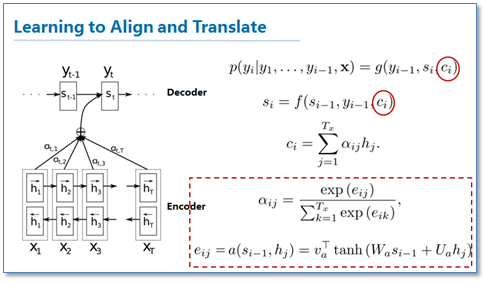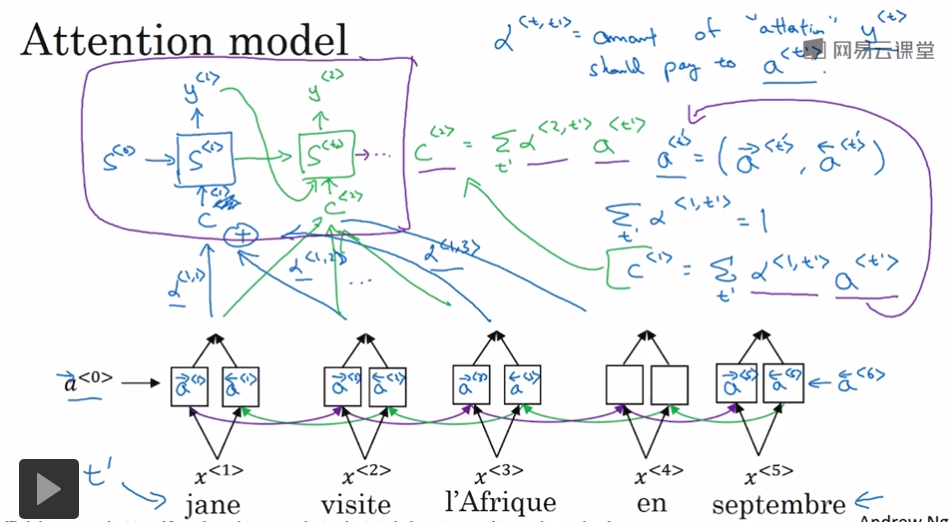如图1图2描述，解码器在解码过程中不使用信息损失较大的final state，而是把encoder每个编码单元的输出都“看”一遍，让模型自己学习如何分配“注意力”，即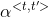，继而求得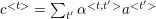，中间涉及到的求取、softmax等细节问题将在下节讲到。

# 2 - attention_wrapper.py

讲解代码前，先将容易引起误解的变量含义说明一下：

•  memory: “记忆”，指encoder的outputs
•  query: decoder当前cell的输入隐藏状态，决定读取memory的哪些部分
tf-1.3.0中，关于attention机制的代码位于tensorflow/contrib/seq2seq/python/ops/attention_wrapper.py文件中，该py文件主要包含4大块：

1) Attention mechanism: 用来实现计算不同类型的attention vector（即context加权和后的向量），包括：

a. class _BaseAttentionMechanism: 所有attention的基类

b. class BahdanauAttention: 论文https://arxiv.org/abs/1409.0473中的实现: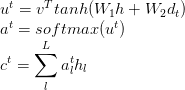c. class LuongAttention: 论文https://arxiv.org/abs/1508.04025中的实现: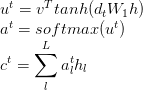d._BaseMonotonicAttentionMechanism，BahdanauMonotonicAttention，LuongMonotonicAttention还未研究，应该跟上述类似

2) AttentionWrapperState类: 继承自namedtuple，类似rnn中的state(LSTMStateTuple)，这里存储了cell_state, attention, time, alignments, alignments_history等信息

3) AttentionWrapper: 将rnn cell与上述attention mechanism封装在一起，从而构建一个带有attention机制的Decoder

4) 公用方法

# 3 - class AttentionWrapper

接下来，BahdanauAttention为例，采用顺叙与插叙方式，以class AttentionWrapper为起点进行详述

def __init__(self,
cell,
attention_mechanism,
attention_layer_size=None,
alignment_history=False,
cell_input_fn=None,
output_attention=True,
initial_cell_state=None,
name=None):
• cellrnn cell实例，可以是单个cell，也可以是多个cell stack后的mutli layer rnn
• attention_mechanism: 上述的attention mechanism的实例，此处以BahdanauAttention为例
• attention_layer_size用来控制我们最后生成的attention是怎么得来的，如果是None，则直接返回对应attention mechanism计算得到的加权和向量；如果不是None，则在调用_compute_attention方法时，得到的加权和向量还会与output进行concat，然后再经过一个线性映射，变成维度为attention_layer_size的向量
• alignment_history主要用于后期的可视化，如果为真，则输出state中alignment_history为TensorArray，记录每个时刻的alignment
• cell_input_fninput送入decoder cell的方式，默认是会将input和上一步计算得到的attention拼接起来送入decoder cell
• output_attention是否返回attention，如果为False则直接返回rnn cell的输出，注意，无论是否为True，每一个时间步的attention都会存储在AttentionWrapperState的一个实例中
• initial_cell_state: 初始状态，此时如果传入，需确保其batch_size与成员函数zero_state所需的参数一致
def __init__(self,
cell,
attention_mechanism,
attention_layer_size=None,
alignment_history=False,
cell_input_fn=None,
output_attention=True,
initial_cell_state=None,
name=None):

super(AttentionWrapper, self).__init__(name=name)
if not rnn_cell_impl._like_rnncell(cell):  # pylint: disable=protected-access
raise TypeError(
"cell must be an RNNCell, saw type: %s" % type(cell).__name__)
if isinstance(attention_mechanism, (list, tuple)):
self._is_multi = True
attention_mechanisms = attention_mechanism
for attention_mechanism in attention_mechanisms:
if not isinstance(attention_mechanism, AttentionMechanism):
raise TypeError(
"attention_mechanism must contain only instances of "
"AttentionMechanism, saw type: %s"
% type(attention_mechanism).__name__)
else: # 此处只考虑self._is_multi为False的情况，即单个attention_mechanism
self._is_multi = False
if not isinstance(attention_mechanism, AttentionMechanism):
raise TypeError(
"attention_mechanism must be an AttentionMechanism or list of "
"multiple AttentionMechanism instances, saw type: %s"
% type(attention_mechanism).__name__)
attention_mechanisms = (attention_mechanism,)

# cell_input_fn默认将attention与input沿最后一维联结，返回当前cell的输入，此处可根据需要对
# lambda函数进行修改，如lambda inputs, attention: attention
if cell_input_fn is None:
cell_input_fn = (
lambda inputs, attention: array_ops.concat([inputs, attention], -1))
else:
if not callable(cell_input_fn):
raise TypeError(
"cell_input_fn must be callable, saw type: %s"
% type(cell_input_fn).__name__)

# attention_layer_size不为None时，以该值为参数定义Dense layer，并作为函数_compute_attention
# 的参数，详见_compute_attention函数
if attention_layer_size is not None:
attention_layer_sizes = tuple(
attention_layer_size
if isinstance(attention_layer_size, (list, tuple))
else (attention_layer_size,))
if len(attention_layer_sizes) != len(attention_mechanisms):
raise ValueError(
"If provided, attention_layer_size must contain exactly one "
"integer per attention_mechanism, saw: %d vs %d"
% (len(attention_layer_sizes), len(attention_mechanisms)))
self._attention_layers = tuple(
layers_core.Dense(
attention_layer_size, name="attention_layer", use_bias=False)
for attention_layer_size in attention_layer_sizes)
self._attention_layer_size = sum(attention_layer_sizes)
else:
self._attention_layers = None
self._attention_layer_size = sum(
attention_mechanism.values.get_shape()[-1].value
for attention_mechanism in attention_mechanisms)

self._cell = cell
self._attention_mechanisms = attention_mechanisms
self._cell_input_fn = cell_input_fn
self._output_attention = output_attention
self._alignment_history = alignment_history
# 如果initial_cell_state为None，则在调用成员函数zero_state时进行初始化，如果不为None，
# 需确保与zero_state的参数batch_size匹配
with ops.name_scope(name, "AttentionWrapperInit"):
if initial_cell_state is None:
self._initial_cell_state = None
else:
final_state_tensor = nest.flatten(initial_cell_state)[-1]
state_batch_size = (
final_state_tensor.shape.value
or array_ops.shape(final_state_tensor))
error_message = (
"When constructing AttentionWrapper %s: " % self._base_name +
"Non-matching batch sizes between the memory "
"(encoder output) and initial_cell_state.  Are you using "
"the BeamSearchDecoder?  You may need to tile your initial state "
"via the tf.contrib.seq2seq.tile_batch function with argument "
"multiple=beam_width.")
with ops.control_dependencies(
self._batch_size_checks(state_batch_size, error_message)):
self._initial_cell_state = nest.map_structure(
lambda s: array_ops.identity(s, name="check_initial_cell_state"),
initial_cell_state)
def zero_state(self, batch_size, dtype):
with ops.name_scope(type(self).__name__ + "ZeroState", values=[batch_size]):
if self._initial_cell_state is not None:
cell_state = self._initial_cell_state
else:
cell_state = self._cell.zero_state(batch_size, dtype)
error_message = (
"When calling zero_state of AttentionWrapper %s: " % self._base_name +
"Non-matching batch sizes between the memory "
"(encoder output) and the requested batch size.  Are you using "
"the BeamSearchDecoder?  If so, make sure your encoder output has "
"been tiled to beam_width via tf.contrib.seq2seq.tile_batch, and "
"the batch_size= argument passed to zero_state is "
"batch_size * beam_width.")
with ops.control_dependencies(
self._batch_size_checks(batch_size, error_message)):
cell_state = nest.map_structure(
lambda s: array_ops.identity(s, name="checked_cell_state"),
cell_state)
return AttentionWrapperState(
cell_state=cell_state,
time=array_ops.zeros([], dtype=dtypes.int32),
attention=_zero_state_tensors(self._attention_layer_size, batch_size,
dtype),
alignments=self._item_or_tuple(
attention_mechanism.initial_alignments(batch_size, dtype)
for attention_mechanism in self._attention_mechanisms),
alignment_history=self._item_or_tuple(
tensor_array_ops.TensorArray(dtype=dtype, size=0,
dynamic_size=True)
if self._alignment_history else ()
for _ in self._attention_mechanisms))

zero_state: 返回AttentionWrapperState实例，作为初始参数

def call(self, inputs, state):
"""Perform a step of attention-wrapped RNN.

- Step 1: Mix the inputs and previous step's attention output via
cell_input_fn.
- Step 2: Call the wrapped cell with this input and its previous state.
- Step 3: Score the cell's output with attention_mechanism.
- Step 4: Calculate the alignments by passing the score through the
normalizer.
- Step 5: Calculate the context vector as the inner product between the
alignments and the attention_mechanism's values (memory).
- Step 6: Calculate the attention output by concatenating the cell output
and context through the attention layer (a linear layer with
attention_layer_size outputs).

Args:
inputs: (Possibly nested tuple of) Tensor, the input at this time step.
state: An instance of AttentionWrapperState containing
tensors from the previous time step.

Returns:
A tuple (attention_or_cell_output, next_state), where:

- attention_or_cell_output depending on output_attention.
- next_state is an instance of AttentionWrapperState
containing the state calculated at this time step.

Raises:
TypeError: If state is not an instance of AttentionWrapperState.
"""
if not isinstance(state, AttentionWrapperState):
raise TypeError("Expected state to be instance of AttentionWrapperState. "

# Step 1: 调用self._cell_input_fn函数，求取cell_inputs
cell_inputs = self._cell_input_fn(inputs, state.attention)
cell_state = state.cell_state
# Step 2: 调用self._cell，求取当前cell的cell_output, next_cell_state
cell_output, next_cell_state = self._cell(cell_inputs, cell_state)

cell_batch_size = (
cell_output.shape.value or array_ops.shape(cell_output))
error_message = (
"When applying AttentionWrapper %s: " % self.name +
"Non-matching batch sizes between the memory "
"(encoder output) and the query (decoder output).  Are you using "
"the BeamSearchDecoder?  You may need to tile your memory input via "
"the tf.contrib.seq2seq.tile_batch function with argument "
"multiple=beam_width.")
with ops.control_dependencies(
self._batch_size_checks(cell_batch_size, error_message)):
cell_output = array_ops.identity(
cell_output, name="checked_cell_output")

if self._is_multi:
previous_alignments = state.alignments
previous_alignment_history = state.alignment_history
else:
previous_alignments = [state.alignments]
previous_alignment_history = [state.alignment_history]

all_alignments = []
all_attentions = []
all_histories = []
# Step 3: 计算当前cell的attention、alignments，详见下文
for i, attention_mechanism in enumerate(self._attention_mechanisms):
attention, alignments = _compute_attention(
attention_mechanism, cell_output, previous_alignments[i],
self._attention_layers[i] if self._attention_layers else None)
alignment_history = previous_alignment_history[i].write(
state.time, alignments) if self._alignment_history else ()

all_alignments.append(alignments)
all_histories.append(alignment_history)
all_attentions.append(attention)

attention = array_ops.concat(all_attentions, 1)
next_state = AttentionWrapperState(
time=state.time + 1,
cell_state=next_cell_state,
attention=attention,
alignments=self._item_or_tuple(all_alignments),
alignment_history=self._item_or_tuple(all_histories))
# attention返回与否，都会保存在next_state中
if self._output_attention:
return attention, next_state
else:
return cell_output, next_state
def _compute_attention(attention_mechanism, cell_output, previous_alignments,
attention_layer):
"""Computes the attention and alignments for a given attention_mechanism."""
# Step 3.1: 计算normalized alignments，shape [batch_size, memory_time]，详见下文
alignments = attention_mechanism(
cell_output, previous_alignments=previous_alignments)
# Step 3.2: 计算attention
# Reshape from [batch_size, memory_time] to [batch_size, 1, memory_time]
expanded_alignments = array_ops.expand_dims(alignments, 1)
# Context is the inner product of alignments and values along the
# memory time dimension.
# alignments shape: [batch_size, 1, memory_time]
# attention_mechanism.values shape is
#   [batch_size, memory_time, attention_mechanism.num_units]
# the batched matmul is over memory_time, so the output shape is
#   [batch_size, 1, attention_mechanism.num_units].
# we then squeeze out the singleton dim.
context = math_ops.matmul(expanded_alignments, attention_mechanism.values)
context = array_ops.squeeze(context, )
# context为真正的attention，如果在构造AttentionWrapper时传入attention_layer_size,
# 内部以此构造attention_layer(Dense layer)，将cell_output、context联接作为输入，
# 则输出attention的shape: [batch_size, attention_layer_size]
if attention_layer is not None:
attention = attention_layer(array_ops.concat([cell_output, context], 1))
else:
attention = context

return attention, alignments

BahdanauAttention包含两部分：W1h + W2dt，详见下文。

# Step 3.1: 计算alignments
class BahdanauAttention(_BaseAttentionMechanism):
This attention has two forms.  The first is Bahdanau attention,
The second is the normalized form.
To enable the second form, construct the object with parameter
normalize=True.
"""
def __init__(self,
num_units,
memory,
memory_sequence_length=None,
normalize=False,
probability_fn=None,
name="BahdanauAttention"):
"""Construct the Attention mechanism.

Args:
num_units: 用以构造query_layer、memory_layer(俩个Dense layer)，也是Decoder cell的
number of hidden units.
memory: ‘记忆’，指Encoder的output，shape [batch_size, max_time, ...].
memory_sequence_length (optional): Encoder输入的真实长度，shape [batch_size],用以
normalize: Python boolean.  Whether to normalize the energy term.
probability_fn: (optional) A callable.  将得分score转换为概率，默认@{tf.nn.softmax}，
其他可选@{tf.contrib.seq2seq.hardmax}，@{tf.contrib.sparsemax.sparsemax}.
Its signature should be: probabilities = probability_fn(score).
name: Name to use when creating ops.
"""
if probability_fn is None:
probability_fn = nn_ops.softmax
wrapped_probability_fn = lambda score, _: probability_fn(score)
# 详见下文
super(BahdanauAttention, self).__init__(
query_layer=layers_core.Dense(
num_units, name="query_layer", use_bias=False),
memory_layer=layers_core.Dense(
num_units, name="memory_layer", use_bias=False),
memory=memory,
probability_fn=wrapped_probability_fn,
memory_sequence_length=memory_sequence_length,
name=name)
self._num_units = num_units
self._normalize = normalize
self._name = name
# Step 3.1.1: 计算alignments之W1h(初始化时已经完成)
class _BaseAttentionMechanism(AttentionMechanism):
"""A base AttentionMechanism class providing common functionality.
Common functionality includes:
1. Storing the query and memory layers.
2. Preprocessing and storing the memory.
"""
def __init__(self,
query_layer,
memory,
probability_fn,
memory_sequence_length=None,
memory_layer=None,
check_inner_dims_defined=True,
name=None):
"""Construct base AttentionMechanism class.
Args:
参数同上
"""
if (query_layer is not None
and not isinstance(query_layer, layers_base.Layer)):
raise TypeError(
"query_layer is not a Layer: %s" % type(query_layer).__name__)
if (memory_layer is not None
and not isinstance(memory_layer, layers_base.Layer)):
raise TypeError(
"memory_layer is not a Layer: %s" % type(memory_layer).__name__)
self._query_layer = query_layer
self._memory_layer = memory_layer
if not callable(probability_fn):
raise TypeError("probability_fn must be callable, saw type: %s" %
type(probability_fn).__name__)
self._probability_fn = lambda score, prev: (  # pylint:disable=g-long-lambda
probability_fn(
prev))
with ops.name_scope(
name, "BaseAttentionMechanismInit", nest.flatten(memory)):
# shape [batch_size, maxlen, num_encoder_units]
self._values = _prepare_memory(
memory, memory_sequence_length,
check_inner_dims_defined=check_inner_dims_defined)
# 此处通过Dense layer计算W1h，并保存在self._keys中，因h在Encoder完成后不在变化，因此该项
# 在初始化时已经计算完成，shape [batch_size, maxlen, num_units]
self._keys = (
self.memory_layer(self._values) if self.memory_layer  # pylint: disable=not-callable
else self._values)
self._batch_size = (
self._keys.shape.value or array_ops.shape(self._keys))
# self._alignments_size = maxlen
self._alignments_size = (self._keys.shape.value or
array_ops.shape(self._keys))
# Step 3.1.1: 计算alignments之W1h
if memory_sequence_length is None:
return score
message = ("All values in memory_sequence_length must greater than zero.")
with ops.control_dependencies(
[check_ops.assert_positive(memory_sequence_length, message=message)]):
memory_sequence_length, maxlen=array_ops.shape(score))
return array_ops.where(score_mask, score, score_mask_values)
# Step 3.1.1: 计算alignments之W1h
def _prepare_memory(memory, memory_sequence_length, check_inner_dims_defined):
"""Convert to tensor and possibly mask memory.
Args:
memory: Tensor, shape: [batch_size, max_time, ...].
memory_sequence_length: int32 Tensor, shaped [batch_size].
check_inner_dims_defined: Python boolean.  If True, the memory
argument's shape is checked to ensure all but the two outermost
dimensions are fully defined.
Returns:
A (possibly masked), checked, new memory.

Raises:
ValueError: If check_inner_dims_defined is True and not
memory.shape[2:].is_fully_defined().
"""
memory = nest.map_structure(
lambda m: ops.convert_to_tensor(m, name="memory"), memory)
if memory_sequence_length is not None:
memory_sequence_length = ops.convert_to_tensor(
memory_sequence_length, name="memory_sequence_length")
if check_inner_dims_defined:
def _check_dims(m):
if not m.get_shape()[2:].is_fully_defined():
raise ValueError("Expected memory %s to have fully defined inner dims, "
"but saw shape: %s" % (m.name, m.get_shape()))
nest.map_structure(_check_dims, memory)
if memory_sequence_length is None:
else:
memory_sequence_length,
maxlen=array_ops.shape(nest.flatten(memory)),
dtype=nest.flatten(memory).dtype)
seq_len_batch_size = (
memory_sequence_length.shape.value
or array_ops.shape(memory_sequence_length))
rank = m.get_shape().ndims
rank = rank if rank is not None else array_ops.rank(m)
extra_ones = array_ops.ones(rank - 2, dtype=dtypes.int32)
m_batch_size = m.shape.value or array_ops.shape(m)
if memory_sequence_length is not None:
message = ("memory_sequence_length and memory tensor batch sizes do not "
"match.")
with ops.control_dependencies([
check_ops.assert_equal(
seq_len_batch_size, m_batch_size, message=message)]):
# reshape seq_len_mask from [batch_size, maxlen] to [batch_size, maxlen, 1,...]，
# 用以broadcast，memory shape [batch_size, maxlen, num_encoder_units]
else:
return m
return nest.map_structure(lambda m: _maybe_mask(m, seq_len_mask), memory)
# Step 3.1.2: 计算W2dt及alignments
# BahdanauAttention: __call__
def __call__(self, query, previous_alignments):
"""Score the query based on the keys and values.
Args:
query: 当前cell的output，shape [batch_size, query_depth].
previous_alignments: Tensor of dtype matching self.values and shape
[batch_size, alignments_size]，(alignments_size is memory's max_time).

Returns:
alignments: Tensor of dtype matching self.values and shape
[batch_size, alignments_size] (alignments_size is memory's max_time).
"""
with variable_scope.variable_scope(None, "bahdanau_attention", [query]):
# 通过Dense layer计算第二项W2dt，该项与当前cell的output有关
processed_query = self.query_layer(query) if self.query_layer else query
# 计算unnormalized score，shape [batch_size, maxlen_of_memory]，详见下文
score = _bahdanau_score(processed_query, self._keys, self._normalize)
# 返回normalized alignments，shape [batch_size, maxlen_of_memory]，score是经过
alignments = self._probability_fn(score, previous_alignments)
return alignments
# Step 3.1: 计算alignments
def _bahdanau_score(processed_query, keys, normalize):

This attention has two forms.  The first is Bhandanau attention.
The second is the normalized form.
To enable the second form, set normalize=True.
Args:
processed_query: Tensor, shape [batch_size, num_units] to compare to keys.
keys: Processed memory, shape [batch_size, max_time, num_units].
normalize: Whether to normalize the score function.

Returns:
A [batch_size, max_time] tensor of unnormalized score values.
"""
dtype = processed_query.dtype
# Get the number of hidden units from the trailing dimension of keys
num_units = keys.shape.value or array_ops.shape(keys)
# Reshape from [batch_size, ...] to [batch_size, 1, ...] for broadcasting.
processed_query = array_ops.expand_dims(processed_query, 1)
v = variable_scope.get_variable(
"attention_v", [num_units], dtype=dtype)
if normalize:
# Scalar used in weight normalization
g = variable_scope.get_variable(
"attention_g", dtype=dtype,
initializer=math.sqrt((1. / num_units)))
# Bias added prior to the nonlinearity
b = variable_scope.get_variable(
"attention_b", [num_units], dtype=dtype,
initializer=init_ops.zeros_initializer())
# normed_v = g * v / ||v||
normed_v = g * v * math_ops.rsqrt(
math_ops.reduce_sum(math_ops.square(v)))
return math_ops.reduce_sum(
normed_v * math_ops.tanh(keys + processed_query + b), )
else:
# keys shape: [batch_size, maxlen, num_units]
# processed_query shape: [batch_size, 1, num_units]
# 返回值shape: [batch_size, maxlen]，unnormalized
return math_ops.reduce_sum(v * math_ops.tanh(keys + processed_query), )

# 4 - Decoder简单应用：

cells=[rnn.LSTMCell(cell_size) for i in range(num_layers)]
mutli_cells = rnn.MultiRNNCell(cells)

attention_mechanism = BahdanauAttention(num_units,
memory=context,
memory_sequence_length=None,
normalize=False,
probability_fn=None,
name="BahdanauAttention")

decoder_cell = AttentionWrapper(cell=mutli_cells,
attention_mechanism=attention_mechanism,
attention_layer_size=None,
alignment_history=True,
output_attention=False,
cell_input_fn=None)

state = decoder_cell.zeros_state(batch_size, tf.float32)
with tf.variable_scope(SCOPE, reuse=tf.AUTO_REUSE):
for i in range(decode_time_steps):
cell_output, state=decoder_cell(decoder_inputs, state)

# References:

 deeplearning.ai Course 5

 https://blog.csdn.net/qsczse943062710/article/details/79539005

 https://xueqiu.com/3426965578/88758188

 https://www.cnblogs.com/robert-dlut/p/5952032.html